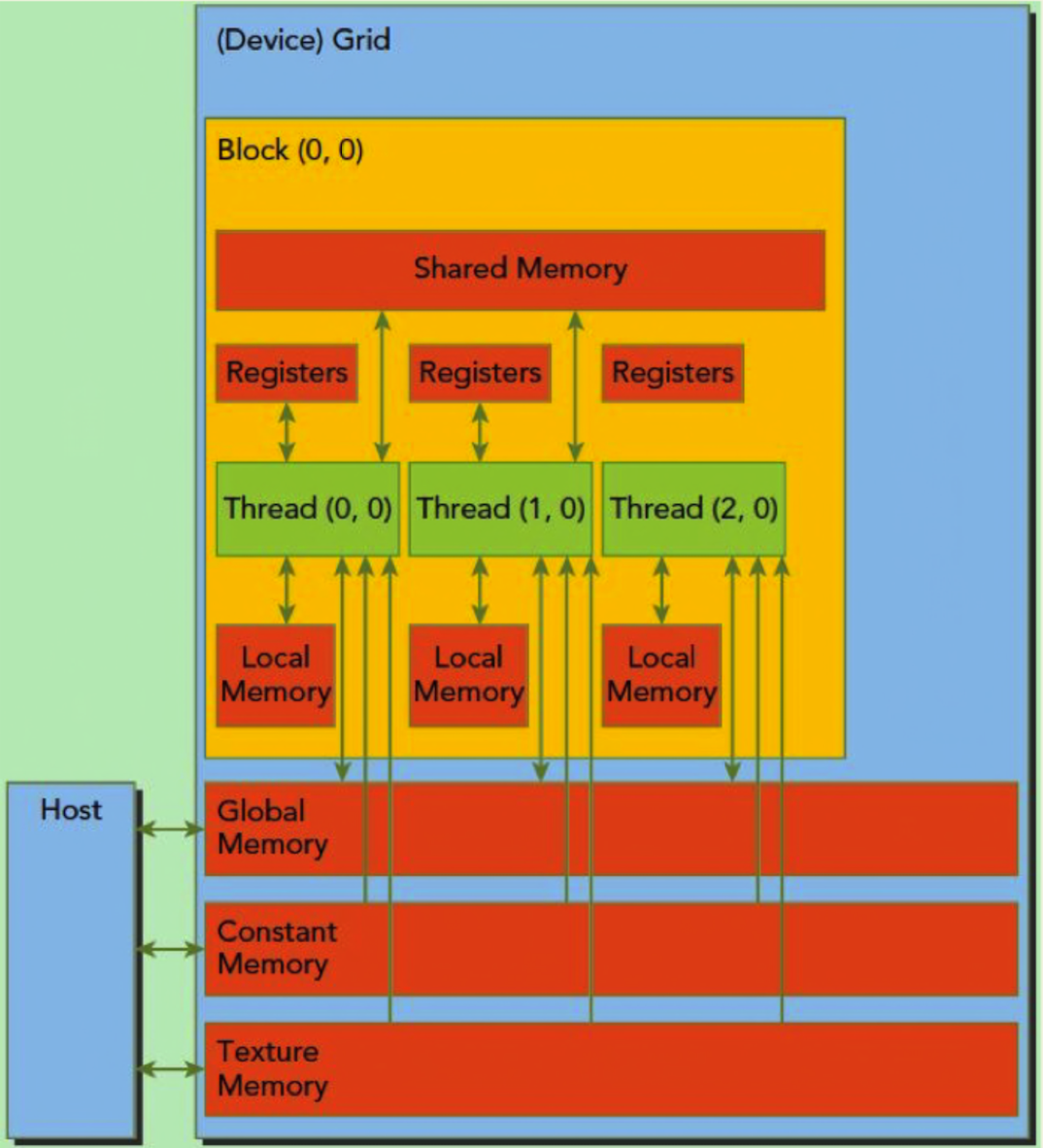# 设备内存// Device code
__global__ void VecAdd(float* A, float* B, float* C, int N) {
int i = blockDim.x * blockIdx.x + threadIdx.x;
if (i < N)
C[i] = A[i] + B[i];
}

// Host code
int main() {
int N = ...;
size_t size = N * sizeof(float);

// Allocate input vectors h_A and h_B in host memory
float* h_A = (float*)malloc(size);
float* h_B = (float*)malloc(size);

// Initialize input vectors
...

// Allocate vectors in device memory
float* d_A;
cudaMalloc(&d_A, size);
float* d_B;
cudaMalloc(&d_B, size);
float* d_C;
cudaMalloc(&d_C, size);

// Copy vectors from host memory to device memory
cudaMemcpy(d_A, h_A, size, cudaMemcpyHostToDevice);
cudaMemcpy(d_B, h_B, size, cudaMemcpyHostToDevice);

// Invoke kernel

// Copy result from device memory to host memory
// h_C contains the result in host memory
cudaMemcpy(h_C, d_C, size, cudaMemcpyDeviceToHost);

// Free device memory
cudaFree(d_A);
cudaFree(d_B);
cudaFree(d_C);

// Free host memory
...
}

// Device code
__global__ void MyKernel(float* devPtr, size_t pitch, int width, int height) {
for (int r = 0; r < height; ++r) {
float* row = (float*)((char*)devPtr + r * pitch);
for (int c = 0; c < width; ++c) {
float element = row[c];
}
}
}

// Host code
int width = 64, height = 64;
float* devPtr;
size_t pitch;
cudaMallocPitch(&devPtr, &pitch, width * sizeof(float), height);
MyKernel<<<100, 512>>>(devPtr, pitch, width, height);

// Device code
__global__ void MyKernel(cudaPitchedPtr devPitchedPtr, int width, int height, int depth) {
char* devPtr = devPitchedPtr.ptr;
size_t pitch = devPitchedPtr.pitch;
size_t slicePitch = pitch * height;
for (int z = 0; z < depth; ++z) {
char* slice = devPtr + z * slicePitch;
for (int y = 0; y < height; ++y) {
float* row = (float*)(slice + y * pitch);
for (int x = 0; x < width; ++x) {
float element = row[x];
}
}
}
}

// Host code
int width = 64, height = 64, depth = 64;
cudaExtent extent = make_cudaExtent(width * sizeof(float), height, depth);
cudaPitchedPtr devPitchedPtr;
cudaMalloc3D(&devPitchedPtr, extent);
MyKernel<<<100, 512>>>(devPitchedPtr, width, height, depth);

## 常量内存

__constant__ float constData;
float data;
cudaMemcpyToSymbol(constData, data, sizeof(data));
cudaMemcpyFromSymbol(data, constData, sizeof(data));

__device__ float devData;
float value = 3.14f;
cudaMemcpyToSymbol(devData, &value, sizeof(float));

__device__ float* devPointer;
float* ptr;
cudaMalloc(&ptr, 256 * sizeof(float));
cudaMemcpyToSymbol(devPointer, &ptr, sizeof(ptr));

# 锁页内存（Page-Locked Host Memory）

• 使用锁页内存后，锁页内存与设备内存之间的数据传输，可以使用流的方式，和内核函数并发执行；
• 使用锁页内存后，可以将锁页内存映射到设备内存上，减少了和设备间的数据拷贝；
• 对于使用前端总线的系统，使用锁页内存可以提升主机端到设备端传输的带宽；如果将锁页内存指定为合并写（write-combining），则可以进一步提高带宽。

## 被映射内存（Mapped Memory）

• 无需在设备上分配内存，也不用显式传输数据；数据传输是在内核需要的时候隐式进行的。
• 无需使用流重叠数据传输和内核执行；数据传输和内核执行自动重叠。

# 统一虚拟地址空间

• 可以通过 cudaPointerGetAttributes()，来确定指针所指的内存处在主机端还是设备端。
• 进行拷贝时，可以将 cudaMemcpy***() 中的 cudaMemcpyKind 参数设置为 cudaMemcpyDefault，去让函数根据指针所处的位置自行判断应该是从哪里拷到哪里。
• 使用 cudaHostAlloc() 分配的锁页内存，自动是 Portable 的，所有支持统一虚拟编址的设备均可访问。cudaHostAlloc() 返回的指针，无需通过 cudaHostGetDevicePointer()，就可以直接被设备端使用。
• 可以通过查询 unifiedAddressing 来查看设备是否支持统一虚拟编址，返回 1 则支持。

# 参考

CUDA C++ Programming Guide

《CUDA C Programming Guide》(《CUDA C 编程指南》)导读

《CUDA 并行程序设计：GPU 编程指南》

CUDA —- Memory Model